# Python 操作 Excel 数据的封装函数

117次阅读  |  发布于6月以前

## 1 . 前期准备

### 1.1. 初识Excel

Microsoft Excel是Microsoft为使用Windows和Apple Macintosh操作系统的电脑编写的一款电子表格软件。

### 1.2. 格式区别

Excel中有xls和xlsx两种格式，它们之间的区别是：

• 文件格式不同。xls是一个特有的二进制格式，其核心结构是复合文档类型的结构，而xlsx的核心结构是XML类型的结构，采用的是基于 XML的压缩方式，使其占用的空间更小。xlsx 中最后一个 x 的意义就在于此。
• 版本不同。xls是Excel2003及以前版本生成的文件格式，而xlsx是Excel2007及以后版本生成的文件格式。
• 兼容性不同。xlsx格式是向下兼容的，可兼容xls格式。

### 1.3. 库的使用

Python自带的模块中有针对xls格式的xlrd和xlwt模块，但这两个库仅仅是针对xls的操作，当我们要操作xlsx格式文件时，则需要使用到openpyxl第三方库。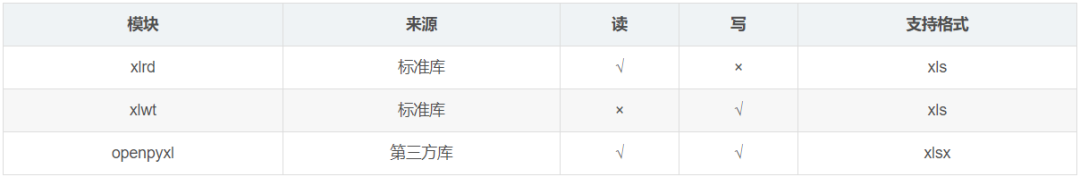### 1.4. 整体思路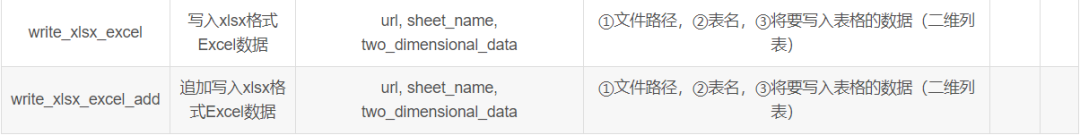## 2. 代码展示

### 2.1. xlz格式

#### 2.1.1. 读取xls格式文件

``````def read_xls_excel(url,index):
'''
读取xls格式文件
参数：
url:文件路径
index：工作表序号（第几个工作表，传入参数从1开始数）
返回：
data:表格中的数据
'''
# 打开指定的工作簿
workbook = xlrd.open_workbook(url)
# 获取工作簿中的所有表格
sheets = workbook.sheet_names()
# 获取工作簿中所有表格中的的第 index 个表格
worksheet = workbook.sheet_by_name(sheets[index-1])
# 定义列表存储表格数据
data = []
# 遍历每一行数据
for i in range(0, worksheet.nrows):
# 定义表格存储每一行数据
da = []
# 遍历每一列数据
for j in range(0, worksheet.ncols):
# 将行数据存储到da列表
da.append(worksheet.cell_value(i, j))
# 存储每一行数据
data.append(da)
# 返回数据
return data``````

#### 2.1.2. 写入xls格式文件

``````def write_xls_excel(url,sheet_name,two_dimensional_data):
'''
写入xls格式文件
参数：
url:文件路径
sheet_name:表名
two_dimensional_data：将要写入表格的数据（二维列表）
'''
# 创建工作簿对象
workbook = xlwt.Workbook()
# 创建工作表对象
# 遍历每一行数据
for i in range(0,len(two_dimensional_data)):
# 遍历每一列数据
for j in range(0,len(two_dimensional_data[i])):
# 写入数据
sheet.write(i,j,two_dimensional_data[i][j])
# 保存
workbook.save(url)
print("写入成功")``````

#### 2.1.3. 追加写入xls格式文件

``````def write_xls_excel_add(url, two_dimensional_data, index):
'''
追加写入xls格式文件
参数：
url:文件路径
two_dimensional_data：将要写入表格的数据（二维列表）
index：指定要追加的表的序号（第几个工作表，传入参数从1开始数）
'''
# 打开指定的工作簿
workbook = xlrd.open_workbook(url)
# 获取工作簿中的所有表格
sheets = workbook.sheet_names()
# 获取指定的表
worksheet = workbook.sheet_by_name(sheets[index-1])
# 获取表格中已存在的数据的行数
rows_old = worksheet.nrows
# 将xlrd对象拷贝转化为xlwt对象
new_workbook = copy(workbook)
# 获取转化后工作簿中的第index个表格
new_worksheet = new_workbook.get_sheet(index-1)
# 遍历每一行数据
for i in range(0, len(two_dimensional_data)):
# 遍历每一列数据
for j in range(0, len(two_dimensional_data[i])):
# 追加写入数据，注意是从i+rows_old行开始写入
new_worksheet.write(i+rows_old, j, two_dimensional_data[i][j])
# 保存工作簿
new_workbook.save(url)
print("追加写入成功")``````

### 2.2. xlsx格式

#### 2.2.1. 读取xlsx格式文件

``````def read_xlsx_excel(url, sheet_name):
'''
读取xlsx格式文件
参数：
url:文件路径
sheet_name:表名
返回：
data:表格中的数据
'''
# 使用openpyxl加载指定路径的Excel文件并得到对应的workbook对象
# 根据指定表名获取表格并得到对应的sheet对象
sheet = workbook[sheet_name]
# 定义列表存储表格数据
data = []
# 遍历表格的每一行
for row in sheet.rows:
# 定义表格存储每一行数据
da = []
# 从每一行中遍历每一个单元格
for cell in row:
# 将行数据存储到da列表
da.append(cell.value)
# 存储每一行数据
data.append(da)
# 返回数据
return data``````

#### 2.2.2. 写入xlsx格式文件

``````def write_xlsx_excel(url, sheet_name, two_dimensional_data):
'''
写入xlsx格式文件
参数：
url:文件路径
sheet_name:表名
two_dimensional_data：将要写入表格的数据（二维列表）
'''
# 创建工作簿对象
workbook = openpyxl.Workbook()
# 创建工作表对象
sheet = workbook.active
# 设置该工作表的名字
sheet.title = sheet_name
# 遍历表格的每一行
for i in range(0, len(two_dimensional_data)):
# 遍历表格的每一列
for j in range(0, len(two_dimensional_data[i])):
# 写入数据（注意openpyxl的行和列是从1开始的，和我们平时的认知是一样的）
sheet.cell(row=i + 1, column=j + 1, value=str(two_dimensional_data[i][j]))
# 保存到指定位置
workbook.save(url)
print("写入成功")
``````

#### 2.2.3. 追加写入xlsx格式文件

``````def write_xlsx_excel_add(url, sheet_name, two_dimensional_data):
'''
追加写入xlsx格式文件
参数：
url:文件路径
sheet_name:表名
two_dimensional_data：将要写入表格的数据（二维列表）
'''
# 使用openpyxl加载指定路径的Excel文件并得到对应的workbook对象
# 根据指定表名获取表格并得到对应的sheet对象
sheet = workbook[sheet_name]
for tdd in two_dimensional_data:
sheet.append(tdd)
# 保存到指定位置
workbook.save(url)
print("追加写入成功")``````

## 3 . 结果测试

### 3.1. 读取测试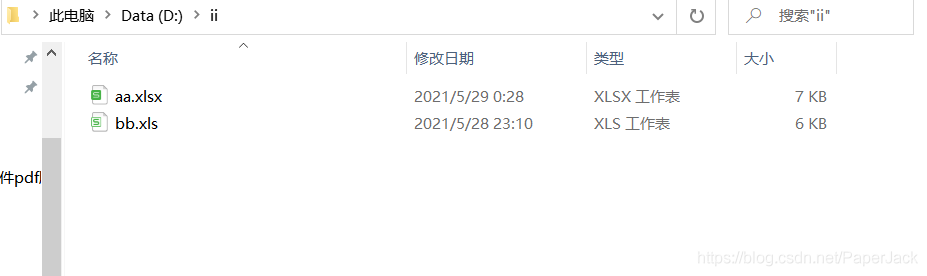其内容如下：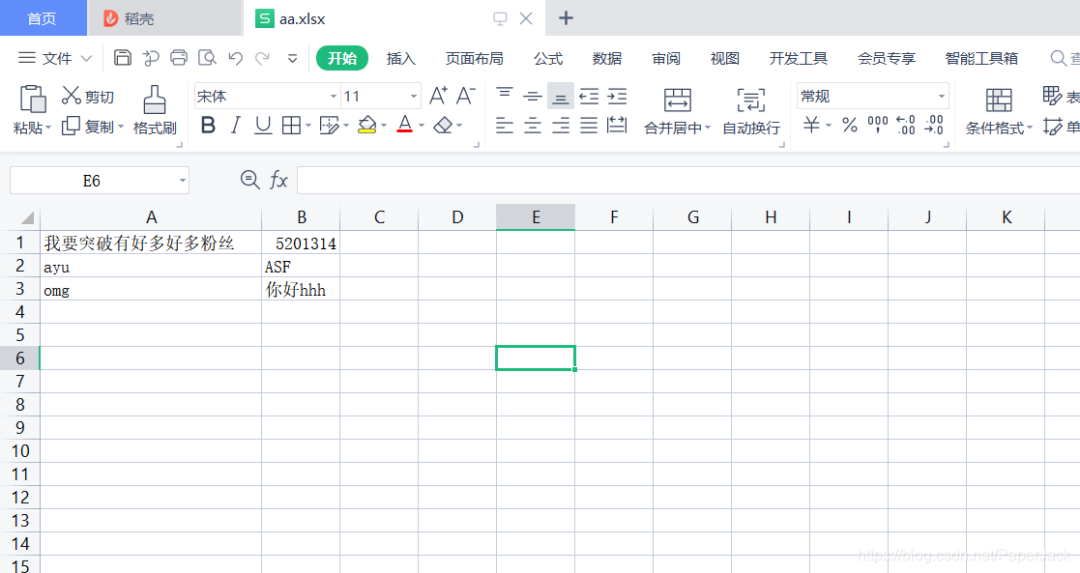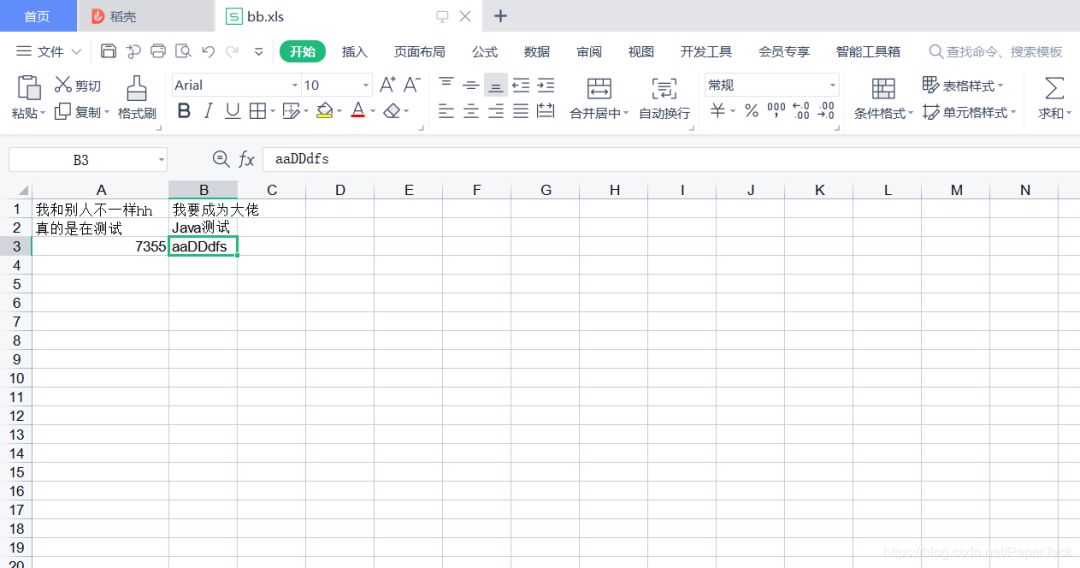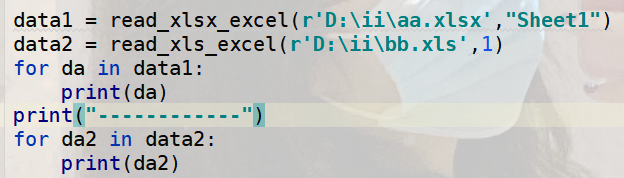输出结果：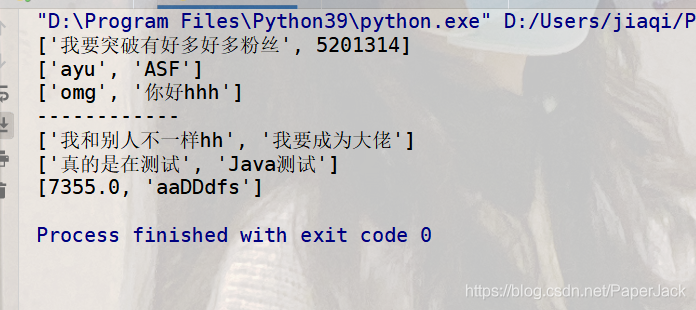结论：表明读取并没有问题！！！接下来测试写入

### 3.2. 写入测试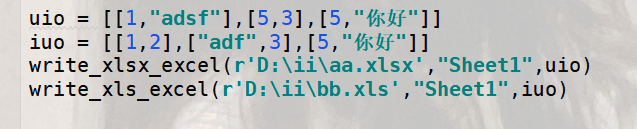结果输出：看到数据被覆盖了！！！接下来在上面修改后的数据的基础上测试追加写入

### 3.3. 追加写入测试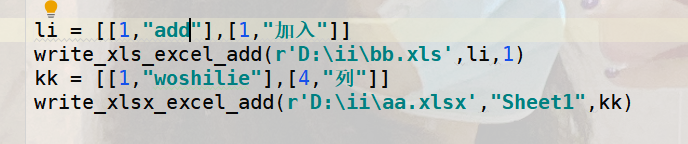结果输出：追加也没有问题！！